# climwin

#### 2019-06-15

climwin is a package in R to help users detect a time period or ‘window’ over which a response variable (usually biological) is sensitive to the environment (usually climate/weather). Periods of climate sensitivity can help inform experimental design, improve species modelling or allow users to more effectively account for climate in statistical modelling. This vignette will give an introduction to the package and its basic features. The advanced vignette provides more detail on the ‘advanced’ features present within the package.

## Why do we need climwin?

The characteristics of an organism are likely to change within a year (e.g. body condition, behaviour), and these will influence the way in which an organism responds to its environment at different points in time. Therefore, when we are interested in studying the effects of climate on some biological response the time period or ‘window’ over which we choose to collect climate data can strongly influence the outcome and interpretation of our results. Yet there has been a tendency in ecological research to focus on a limited number of windows, often chosen arbitrarily. For example, there has been a strong focus on the biological impacts of average spring conditions.

Without a critical comparison of different possible windows, we limit our ability to make meaningful conclusions from our research. If a biological trait displays no response to an arbitrarily chosen climate window it is difficult to determine if this is evidence of insensitivity to climate or whether the choice of time period is flawed. Even when we find a relationship between climate and the biological response, we cannot be sure that we have selected the period where the trait is most sensitive. Therefore, there is a need for a more effective method through which we can select our sampling period.

Realistically, manually testing and comparing all possible windows can be difficult and time consuming. With climwin we hope to overcome this problem by employing exploratory approaches to test and compare the effects of all possible climate windows. This will remove the need to arbitrarily select climate windows, and will consequently improve our research outcomes and conclusions. Below we will outline the basic features of the climwin package, including how to carry out and interpret a climate window analysis.

## slidingwin: The base function

slidingwin is the main function of the climwin package. It uses a sliding window approach to investigate all possible windows and compares them using values of AICc.

### Under the hood

Let’s imagine we have a dataset containing two variables: chick mass at hatching and date of measurement. Chick mass is likely to be measured at different dates due to biological differences (e.g. hatching date, incubation time), therefore our dataset will look something like this:

Date Mass (g)
04/06/2015 120
05/06/2015 123
07/06/2015 110
07/06/2014 140
08/06/2014 138

We want to understand the relationship between temperature and chick mass, so we also have a second dataset containing daily temperature data.

Date Temperature
01/06/2015 15
02/06/2015 16
03/06/2015 12
04/06/2014 18
05/06/2014 20
06/06/2014 23
07/06/2014 21
08/06/2014 27

As is often the case, we have no prior knowledge to help us select the best climate window. To overcome this issue, slidingwin will test all possible windows for us. First, lets imagine we start by testing the effect of average temperature 1-2 days before hatching. slidingwin will calculate the corresponding average temperature for each biological record and create a new combined dataset.

Date Mass (g) Temperature [1 - 2 days]
04/06/2015 120 14
05/06/2015 123 15
07/06/2015 110 21.5
07/06/2014 140 21.5
08/06/2014 138 22

Now that we have this new dataset, we can test the relationship between mass and temperature and determine an AICc value . Let’s use a basic linear model:

lm(Mass ~ Temperature[1 - 2 days])

We’ve tested the relationship between temperature and chick mass in one climate window, but we don’t yet know how this window compares to others. slidingwin will go back and carry out the same process on all other windows and generate a list of AICc values. These values will then be compared to the AICc value of a null model (a model containing no climate). This new value of $$\Delta AICc$$ allows us to not only compare different climate windows with one another but also determine how well each climate window actually explains the biological data. Our final outcome can be seen below:

Window Model AICc Null model AICc Difference
2 - 4 days 1006 1026 20
1 - 3 days 1015 1026 11
1 - 2 days 1019 1026 7
4 - 5 days 1020 1026 6

With this simple comparison, we can see that the strength of the window 2 - 4 days before hatching is not only better than a model with no climate (i.e. the value of AICc is 20 units smaller), but also better than other tested climate windows (i.e. the AICc value is 9 units smaller than the next best window). In this case, we would conclude that chick mass was most strongly influenced by average temperature 2 - 4 days before hatching.

While this example is simplistic, it provides insight into the method behind slidingwin. In practice, slidingwin can be applied to large datasets testing thousands of possible windows, streamlining what would otherwise be a difficult and time consuming process.

### Getting started

Now that we understand how slidingwin works, how do we go about using it? As with our simple example above, it is first necessary to create two separate datasets for the climate and biological data. These two datasets will be the basis for the climate window analysis.

To start using slidingwin it’s important to first understand the basic parameters. Below, we will discuss the parameters required to run a basic sliding window analysis. More ‘advanced’ parameters are discussed in the advanced vignette.

For this example, we will use the Mass and MassClimate datasets included with the climwin package.

xvar

To begin, we need to determine the predictor variable which we want to study. The parameter ‘xvar’ is a list object containing our variables of interest (e.g. Temperature, Rainfall). While we will focus here on climate, it is possible to apply our slidingwin methodology with non-climatic predictors (e.g. NDVI).

xvar = list(Temp = MassClimate$Temperature) cdate/bdate Once we have established our predictor variable, we next need to tell slidingwin the location of our date data. These two parameters contain the date variable for both the climate dataset (‘cdate’) and biological dataset (‘bdate’). N.B. These date variables must be stored in a dd/mm/yyyy format. cdate = MassClimate$Date

bdate = Mass$Date baseline The parameter ‘baseline’ determines the model structure for the null model. The structure of the baseline model is highly versatile, with the potential to use many different model functions in R (lm, lmer, lme, glm, glmer, coxph), include covariates, random effects and weights. Here, we will use a basic linear model. baseline = lm(Mass ~ 1, data = Mass) Climate data from each tested climate window will be added as a predictor to this baseline model, to allow for a direct comparison between the null model and each climate window. cinterval Ideally, our predictive data will be available at a resolution of days. However in some circumstances this may not be possible. With this in mind, cinterval allows users to adjust the resolution of analysis to days, weeks or months. Note that the choice of ‘cinterval’ will impact our interpretation of the parameter ‘range’ (see below). In our current example, daily data is available and so a resolution of days will be used. cinterval = "day" range While our above example used a small dataset and tested a limited number of climate windows, the number of tested climate windows can be much larger, set by the parameter ‘range’. This parameter contains two values which set the upper and lower limit for tested climate windows respectively. The values of range will correspond to the resolution chosen using the parameter ‘cinterval’. In this example we are interested in testing all possible climate windows anywhere up to 150 days before the biological record. range = c(150, 0) type There are two distinct ways to carry out a sliding window analysis. In our simple example of chick mass above we considered ‘relative’ climate windows, where the placement of the window will vary depending on the time of the biological response (e.g. 1-2 days before hatching). These relative windows assume that each individual may have the same relative response to climate, but the exact calendar dates will vary between individuals. Alternatively, there may be situations where we expect all individuals to respond to the same climatic period (e.g. average April temperature). In this case, we assume that all climate windows will be calculated from an ‘absolute’ start date which will act as day 0. In this case, the additional parameter ‘refday’ will set the day and month of day 0 for the climate window analysis. In this example, we will test for absolute climate windows using a starting date of May 20th. For more details on different types of climate window analyses see  and  type = "absolute" refday = c(20, 5) stat While we now have the ability to extract climate data for all possible climate windows, the aggregate statistic with which we analyse this data may also influence our results. Most commonly, we consider the mean value of climate within each window, yet it may be more appropriate to consider other possible aggregate statistics, such as maximum, minimum or slope [3, 4]. The parameter stat allows users to select the aggregate statistic of their choice. stat = "mean" func Although the relationship between climate and our biological response may often be linear, there is a potential for more complex relationships. The parameter ‘func’ allows users to select from a range of possible relationships, including linear (“lin”), quadratic (“quad”), cubic (“cub”), logarithmic (“log”) and inverse (“inv”). func = "lin" N.B climwin also allows for more complex model structures which require more detailed user input. These are outlined in our advanced vignette. ### The finished product After choosing each of our parameter values, we can finally input our choices into the slidingwin function. The below slidingwin syntax will test the linear effect of mean temperature on chick mass across all windows between 0 and 150 days before May 20th. NOTE: Analysis with climwin can require large amounts of computational power. Please be patient with the results. library(climwin) MassWin <- slidingwin(xvar = list(Temp = MassClimate$Temp),
cdate = MassClimate$Date, bdate = Mass$Date,
baseline = lm(Mass ~ 1, data = Mass),
cinterval = "day",
range = c(150, 0),
type = "absolute", refday = c(20, 05),
stat = "mean",
func = "lin")

The product of our climate window analysis is a list object, here we’ve called it MassWin.

The object MassWin has three key components.

• Dataset: is an ordered data frame summarising the results of all tested climate windows. The climate windows are ordered by $$\Delta AICc$$ (the difference between the AICc of each model and the null model). Note, when calling the dataset we need to specify that we’re interested in the dataset from the first list item (i.e. []). As we will discuss below, it is possible to test multiple combinations of parameter levels (e.g. climate variables), which will output multiple list items.
    head(MassWin[]$Dataset) deltaAICc WindowOpen WindowClose ModelBeta ModWeight -64.81496 72 15 -4.481257 0.0268355 -64.55352 72 14 -4.485161 0.0235472 -64.53157 73 15 -4.510254 0.0232902 -64.40163 73 14 -4.517427 0.0218251 -64.30387 72 13 -4.500146 0.0207839 -64.20857 73 13 -4.533436 0.0198168 As we can see above, the best climate window is 72 - 15 days before May 20th, equivalent to temperature between March 9th and May 5th. N.B. As can be seen in the model weights (column ModWeight), there is uncertainty in the exact best model. The window of 72 - 15 days should be taken as the best representative of a broad period of sensitivity. In other words, chick mass responds to temperature around a period of 72 - 15 days before hatching. This uncertainty in the best climate window is accounted for somewhat by using the more complex weightwin approach, outlined in the advanced vignette. • BestModel: is a model object showing the relationship between temperature and mass within the strongest climate window.  MassWin[]$BestModel
Call:
lm(formula = Yvar ~ climate, data = modeldat)

Coefficients:
(Intercept)      climate
163.544       -4.481  
• BestModelData: is a data frame containing the raw climate and biological data used to fit BestModel.
    head(MassWin[]$BestModelData) Yvar climate 140 6.068966 138 6.160345 136 6.781034 135 6.877586 134 6.713793 134 6.120690 ## Accounting for over-fitting With the slidingwin code above, we have compared all possible climate windows for our Mass dataset. However, one danger of the exploratory approach that we employ is that there is a risk of detecting seemingly suitable climate windows simply by statistical chance. In other words, if we fit enough climate windows one of them will eventually look good. To overcome this concern, we include a method to account for over-fitting within the climwin package using the functions randwin and pvalue. These functions are required to make conclusions using climwin. ### randwin The function randwin allows users to re-run their climate window analyses on a dataset where any true climate signal has been removed. The date information in the original dataset is randomly rearranged to remove any association between climate and the biological response. By running slidingwin on this new randomised dataset we can determine the likelihood of finding our original result by random chance. The syntax of randwin is mostly identical to that of slidingwin except for the inclusion of the ‘repeats’ argument. This allows the user to decide how many times they want to randomise the order of the data and re-run the slidingwin analysis. In this scenario we will run 5 repeats to limit computational time; however, it should be noted that running more repeats is better to more accurately account for over-fitting. repeats = 5 NOTE: all other arguments should be consistent between slidingwin and randwin so that results are comparable. MassRand <- randwin(repeats = 5, xvar = list(Temp = MassClimate$Temp),
cdate = MassClimate$Date, bdate = Mass$Date,
baseline = lm(Mass ~ 1, data = Mass),
cinterval = "day",
range = c(150, 0),
type = "absolute", refday = c(20, 05),
stat = "mean",
func = "lin")

The output of the randwin is a list object with one item, here we have called in ‘MassRand’.

MassRand[]
deltaAICc WindowOpen WindowClose ModelBeta ModWeight
-7.381971 43 43 -1.013629 0.0057114
-2.480037 10 9 0.632130 0.0006934
-11.42487 121 118 1.022294 0.0268391
-7.473806 9 7 -0.865967 0.0046362
-11.61841 50 14 -2.338881 0.0048511

Rows in the randwin output show each of the top models from the 5 repeats used. We can see that the $$\Delta AICc$$ values from the randwin output are much larger (i.e. less negative) than those in our original slidingwin analysis, suggesting that the large negative values we observed in our slidingwin analysis are unlikely to have occurred by chance. However, to make this conclusion with more certainty we need to use the pvalue function.

### pvalue

Now that we have results from both slidingwin and randwin we can estimate how likely our observed result would be at random using pvalue. This function has four important arguments:

dataset

The dataset output from our slidingwin analysis.

dataset = MassWin[]$Dataset datasetrand The dataset output from our randwin analysis. datasetrand = MassRand[] metric pvalue includes two methods that can be used to account for over-fitting: • Where the number of repeats used in randwin are high (100+) we can use the metric “AIC”. With a high number of repeats we can confidently determine the distribution of $$\Delta AICc$$ values we would expect in a dataset with no climate signal present, we can then determine how likely our observed $$\Delta AICc$$ result from slidingwin would be in this distribution. • When using large datasets, running 100+ repeats can take large amounts of computational time. When the number of repeats is limited, we use the metric “C”. This estimates the distribution of $$\Delta AICc$$ using the information available from the limited number of repeats. In this scenario, with only 5 repeats, we will use the “C” metric. For more information on these metrics and their effectiveness see . metric = "C" sample.size The number of years of data used in the analysis. This is required when using the “C” metric. In our example, we have 47 years of data. sample.size = 47 With these arguments set we can now run the pvalue function. pvalue(dataset = MassWin[]$Dataset, datasetrand = MassRand[], metric = "C", sample.size = 47)
1.94e-05

The output of the pvalue function is very small (<0.005). This can give us confidence that our original slidingwin result is unlikely to be an issue of overfitting. It should be noted that we do not advocate for a prescribed cut-off for pvalue results (e.g. 0.05). Instead, it is better to use the result from pvalue as a measure of confidence in our observed result.

We can visualise this result using the plothist function. This gives a histogram of the $$\Delta AICc$$ values extracted from randwin and a dashed line to show the $$\Delta AICc$$ of the observed result from slidingwin.

As we can see below, the value of $$\Delta AICc$$ from the real data (dashed line) is very different to those values obtained from randomised data. At the top of the graph we can see the outcome of pvalue calculated using both the “AIC” and “C” metric.

NOTE: For plotting examples we save the dataset from ‘MassWin’ (calculated above) as a new object ‘MassOutput’. i.e. MassOutput <- MassWin[]$Dataset. Similarly, the output from randwin is saved as MassRand. i.e. MassRand <- MassRand[] plothist(dataset = MassOutput, datasetrand = MassRand) ## Warning in plothist(dataset = MassOutput, datasetrand = MassRand): ## PDeltaAICc may be unreliable with so few randomisations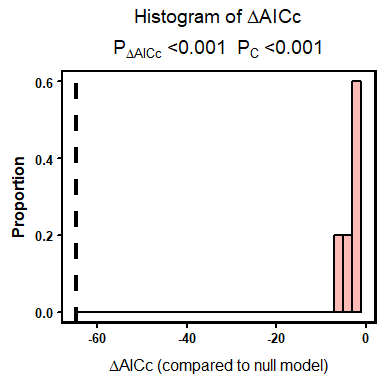## Visualising our data Now that we have confidence in our slidingwin output, we can visualise the results. We have designed 5 plotting options that allow users to visualise their climate window data (plus the plothist function described above). In addition to randwin/pvalue these plots can also be useful to assess confidence in a given result. Below we will discuss each plot in detail. ### Plot $$\Delta AICc$$ values As a first step, we can look at the distribution of $$\Delta AICc$$ values across all tested climate windows. In the below plot, blue regions represent climate windows with limited strength (AICc values similar to the null model) while red regions show strong windows. In our example, we can see an obvious region of strong windows around the left of the graph corresponding to the region around the best window. plotdelta(dataset = MassOutput)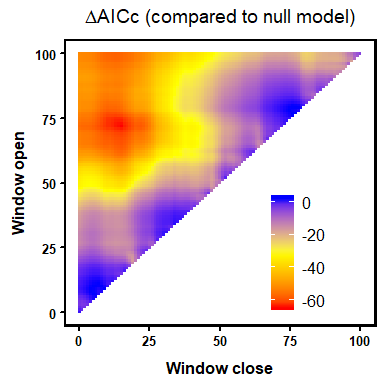It’s worth noting that some biological data may exhibit multiple periods of sensitivity (e.g. long-lag and short-lag ). These can be detected using the plotdelta function. ### Plot model weights Another way to visualise the $$\Delta AICc$$ data is through the use of model weights . In short, the higher a model weight value the greater confidence we have that this model is the true ‘best’ model. While our top climate window is the most likely single window to explain variation in the data, our confidence that this top window represents the true ‘best’ model may still be low. If we sum the weights of multiple models, we can now be more confident that we encompass the true best model, but we are less sure of its exact location. In our model weights plot we shade all models that make up the top 95% of model weights (the 95% confidence set). Therefore, we can be 95% confident that the true ‘best’ model falls within the shaded region. In our example using the Mass dataset, we can see that the top 95% of model weights falls within a small region roughly corresponding to the peak seen in $$\Delta AICc$$ above. In fact, we can be 95% confident that the best climate window falls within only 4% of the total fitted models. This gives us further confidence in our results. plotweights(dataset = MassOutput)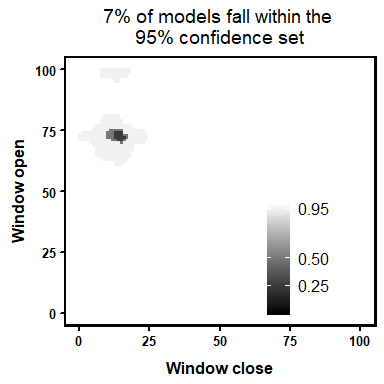### Plot model coefficients While we are often interested in estimating the location of the best climate window, we may also be interested in estimating the relationship between climate and our biological response. The plotbeta function generates a similar colour plot to that used above to model $$\Delta AICc$$, which shows the spread of model coefficients across all fitted climate windows. In the below plot, we can see that windows around our best model show a negative relationship between temperature and mass (red), while other models show little response (blue). plotbetas(dataset = MassOutput)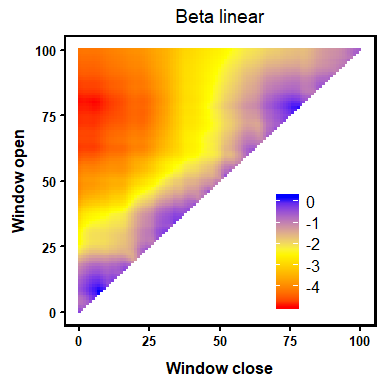### Median window To represent the model weight plot in a different way, plotwin shows boxplots of the start and end point of all climate windows that make up the 95% confidence set. The values above each boxplot represent the median start and end time for these models. In the below example, the median start and end point of the top models corresponds almost exactly with our best window determined using $$\Delta AICc$$. In situations where model weights are more dispersed such a match would be less likely. These median values can also be determined with the function medwin. plotwin(dataset = MassOutput)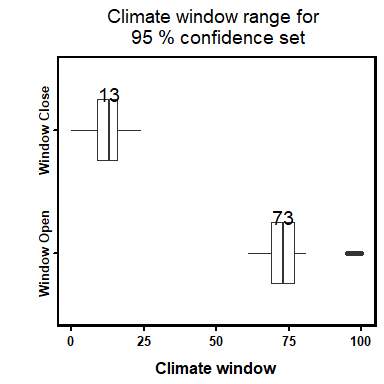### Best model plot Although the above plots and pvalue output give us strong confidence that our results represent a real period of climate sensitivity, we have not considered how well climate within this period actually explains variation in the biological data. Although temperature in March and April explains variation in mass better than at other points in time, temperature over this period may still not explain variation in the biological data that well. Using the plotbest function, we can plot the predictions of our best model over the biological data. Examining this plot can help us determine whether it may be more appropriate to test alternative relationships of climate (e.g. quadratic or cubic) by adjusting the parameter func or to identify outliers. The BestModel object can often be lost when we start a new R session. To overcome this issue, the singlewin function allows you to generate BestModel and BestModelData for a single climate window (e.g. the best window from a previous analysis). MassSingle <- singlewin(xvar = list(Temp = MassClimate$Temp),
cdate = MassClimate$Date, bdate = Mass$Date,
baseline = lm(Mass ~ 1, data = Mass),
cinterval = "day",
range = c(72, 15),
type = "absolute", refday = c(20, 5),
stat = "mean",
func = "lin")

NOTE: In the singlewin function the values of range now equate to the start and end time of the single window, rather than the range over which multiple windows will be tested.

We can use the output from the singlewin function to plot the best data.

plotbest(dataset = MassOutput,
bestmodel = MassSingle$BestModel, bestmodeldata = MassSingle$BestModelData)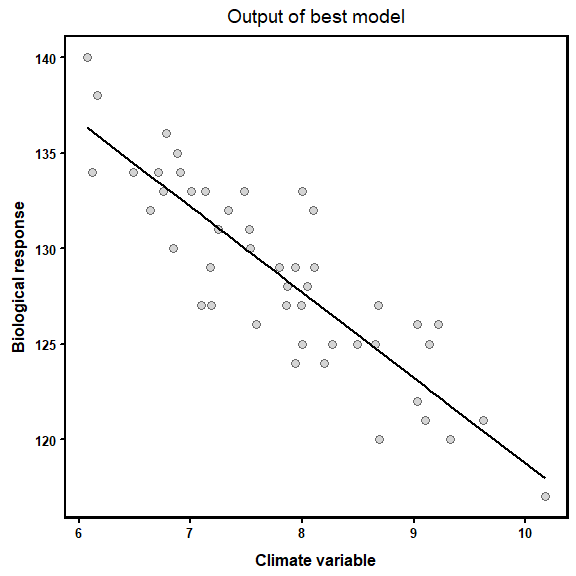### The plotall function

While each of these plots provide useful information for the user, they are best viewed in conjunction. The plotall function allows users to create an array of all graphics to contrast and compare the different information they provide.

plotall(dataset = MassOutput,
datasetrand = MassRand,
bestmodel = MassSingle$BestModel, bestmodeldata = MassSingle$BestModelData)
## Warning in plothist(dataset = dataset, datasetrand = datasetrand):
## PDeltaAICc may be unreliable with so few randomisations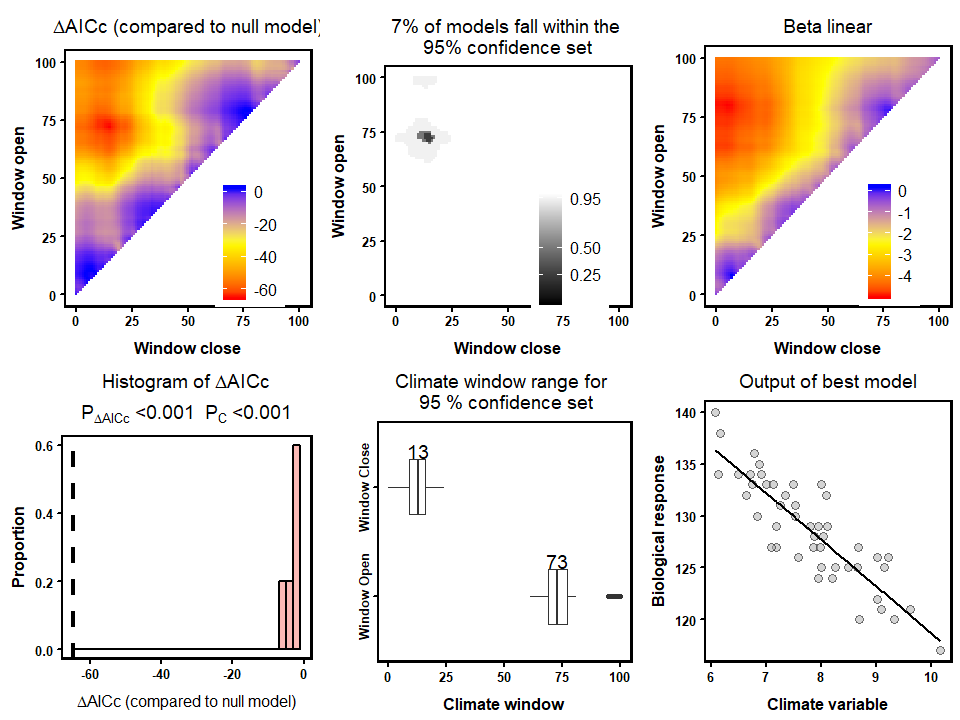## Testing multiple parameter combinations

In the above examples we have been investigating one combination of slidingwin parameters. However, there may be situations where users wish to test different parameter combinations. For example, we may be interested in looking at both the linear and quadratic relationship of temperature or consider investigating the effects of both average and maximum temperature.

For ease of use, slidingwin allows users to test these different combinations with a single function. To take the above examples, we can test both linear and quadratic relationships

func = c("lin", "quad")

and consider both maximum and mean temperature values.

stat = c("max", "mean")

So our final function would be:

MassWin2 <- slidingwin(xvar = list(Temp = MassClimate$Temp), cdate = MassClimate$Date,
bdate = Mass$Date, baseline = lm(Mass ~ 1, data = Mass), cinterval = "day", range = c(150, 0), type = "absolute", refday = c(20, 5), stat = c("max", "mean"), func = c("lin", "quad")) To view all the tested combinations we can call the combos object MassWin2$combos
Climate Type Stat func
1 Temp fixed max lin
2 Temp fixed mean lin
3 Temp fixed max quad
4 Temp fixed mean quad

Using this combos list, we can extract information on each of our tested combinations, as each row in the combos table corresponds to a list item in our object MassWin2. For example, the BestModel object of the quadratic relationship using maximum temperature (3rd row in the above table) would be called using the following code:

MassWin2[]\$BestModel
Call:
lm(formula = Yvar ~ climate + I(climate^2), data = modeldat)

Coefficients:
(Intercept)       climate  I(climate^2)
139.39170      -1.33767       0.03332

This covers the basic functions of climwin. For more advanced features see our extra vignette ‘advanced_climwin’. To ask additional questions or report bugs please e-mail:

or visit our google group.